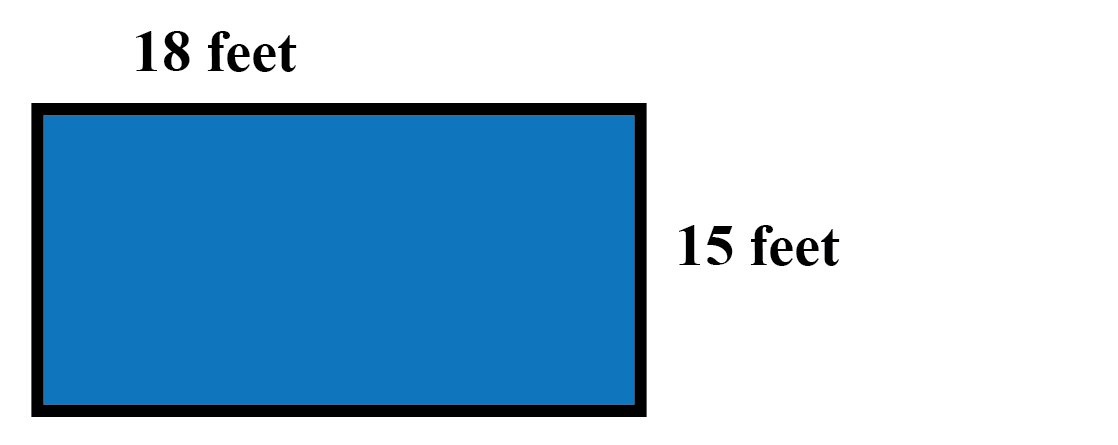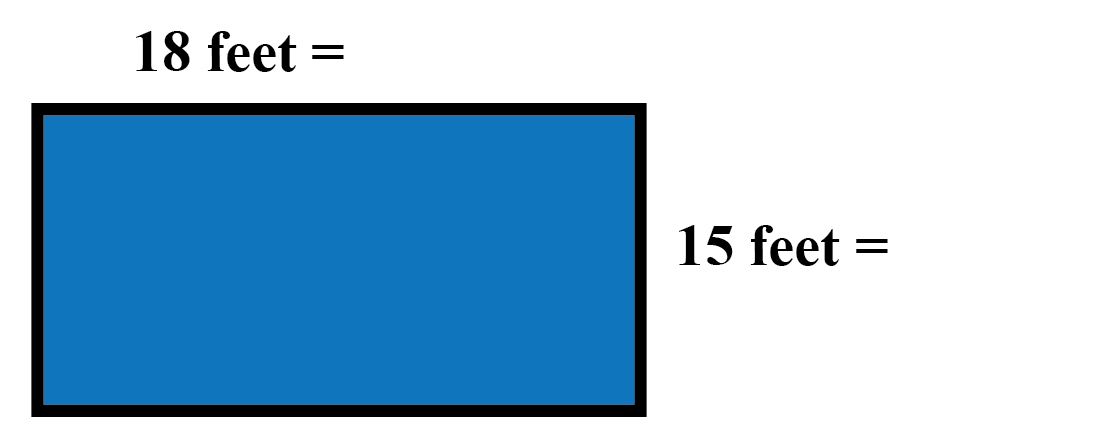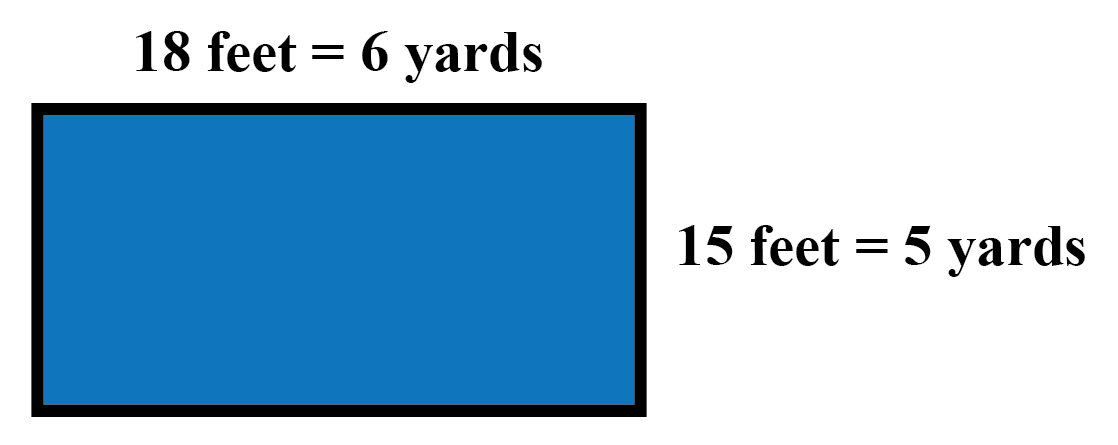### Home > CCAA > Chapter 4 Unit 3 > Lesson CC2: 4.1.1 > Problem4-8

4-8.

Tina’s rectangular living-room floor measures $15$ feet by $18$ feet.1. How many square feet of carpet will Tina need to cover the entire floor?

If it helps, draw a diagram. Find the area of the rectangle that represents the floor. A rectangle labeled, length, 18 feet, and width, 15 feet.﻿

270 square feet

2. The carpet Tina likes is sold by the square yard. How many square yards will she need? ($1 \text{ yard}=3\text{ feet}$)

There are two ways to solve part (b). You can take your answer from part (a) and divide it by the number of square feet
in a square yard, or you can convert the dimensions of the room into yards and then multiply.

If you use the first method, remember to convert your units properly. A square yard is $3$ feet by $3$ feet, or $9$ square feet. The same rectangle labeled, 18 feet = 6 yards, and width labeled, 15 feet = 5 yards.